﻿ True pictures of photoelectric effects and Coulomb force.

# Truth of Electromagnetic fields and waves

## " Photon exchange = Coulomb force "  is unrealistic.

### [ Virtual photon in Feynman QED "disobeys" special relativity ! ]

(Fig.1)   "Photon" is just abstract math symbol (= a ), without physical shape.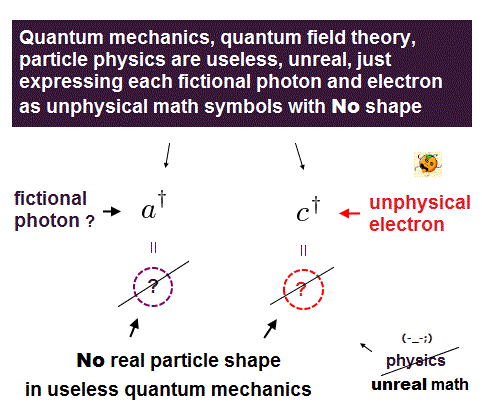Quantum mechanics claims that the classical Coulomb force is caused by photon exchange.
But clear mechanisms of "attractive" and "repulsive" Coulomb forces remain unknown.

In fact, this photon causing Coulomb force is virtual photon, so NOT real ( see this, this, this ).
Surprisingly, this virtual photon is superluminal tachyon, violating Einstein's relativity ( see this, this ).

By the way, what is the physical shape and size of a single photon ?
Unfortunately, quantum mechanics cannot answer this basic question.

In QED, these photons must be described using abstract Feynman diagram.
This Feynman QED has NO ability to show photon's clear picture at all.

All quantum mechanics can show is meaningless math symbols ( see this, this p.3 ).
It contains only rough actions, "a photon is created (= a )", or "annihilated (= a )". That's all, NOT physics.

## Photoelectric effect = "photon" is mind-control by textbooks.

### [ Photoeletric effect related to light "frequency" (= f ) proves light is "wave" ! ]

(Fig.2)   Light frequency (= f ) equal to c/λ (= light wavelength ) proves "Wave" !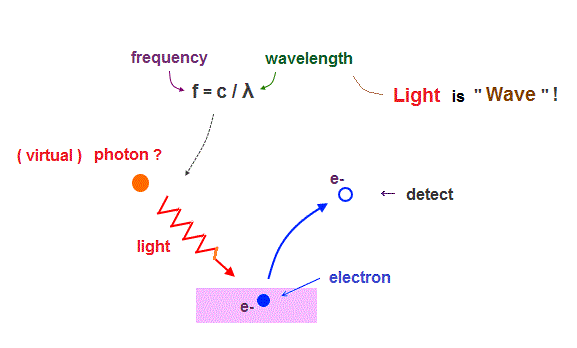Though almost all textbooks say photoelectric effect proposed by Einstein proved particle nature of "photon", this far-fetched interpretation is clearly "brainwashing" by textbooks.

Electrons are "emitted" from metals, when illuminated by light of higher frequency than some threshold, irrelevant to its intensity. So they ( this, this, this ) affirmed that light is "particle".

But as you know, light frequency (= f ) is equal to c/λ (= wavelength ). See this site.
"Frequency" and "wavelength" clearly mean "wave" ( NOT particle ! ) nature of light.

So, the idea "photoelectric effect = light frequency = photon " is one of mind-controls by textbooks and media, like Bohr's accelerating electron ( though it does NOT lose energy ).

In fact, Lamb (= Nobel winner ) argued that the photoelectric effect happens without photon.

Imaginary "photon" is necessary for quantum field theory such as Higgs and string theory.
But these photon's operators (= a, this, this p.5 ) are just abstract math symbols with NO realistic shapes.

## How Big is a photon ? ← Shut up ! ← NOT science.

### [ There is "1000 meter" wavelength light.  A single photon is so Big !? ]

(Fig.3)   The wavelength of radio wave (= one of lights = photon ? ) is 1000 meter !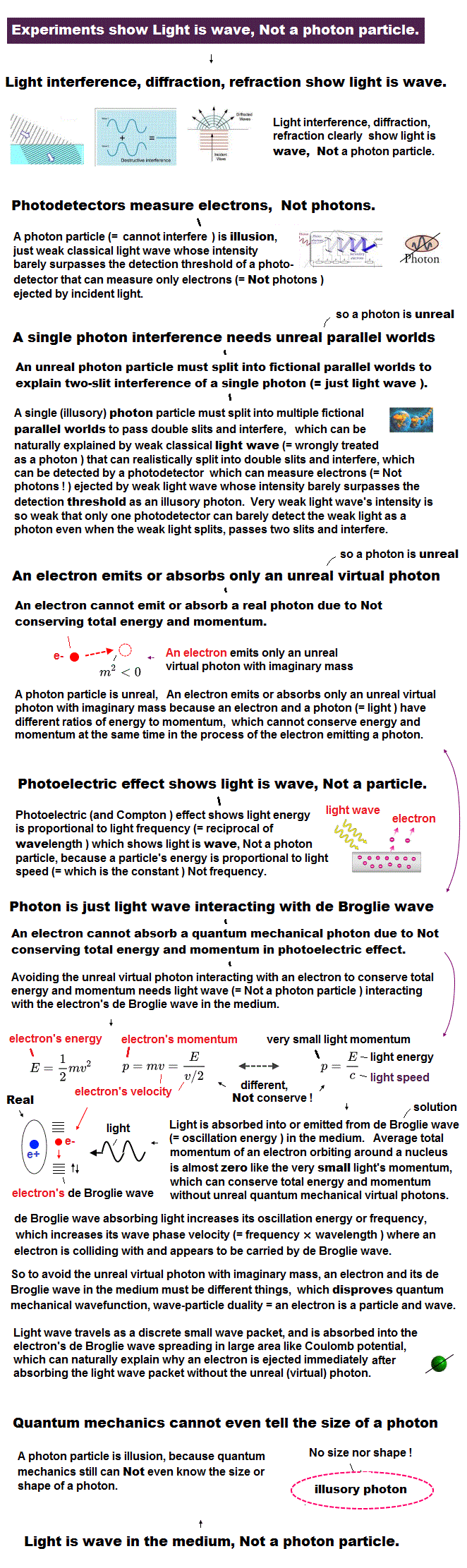Though quantum mechanics claims that light is a photon, the existence of this photon is very unrealistic.  The radio wave is one of lights with long wavelength ( > 1000 meters ! ).

As shown in this, this, low-frequency lights have wavelengths from 1 km to 100000 km !
This means the size of a single photon in these radio waves is as Big as 100000 km ?

If a photon is so Big, we can easily divide a photon into two parts, which means a photon is NOT an elementary particle.  So, a photon is just "media-hype", as shown in clear discrepancy between NBC and insidescience.

Though the media ( this, this ) and universities routinely use the word "photon", they cannot answer "what size and shape of a photon ?". So vague photon represents " NOT asking" quantum mechanics.

Some say a photon has NO size ( this, this ), some say a photon is point-like. But thinking commonsensically, it is impossible that light with 1000 meter wavelength is a point-like particle.

## "Interference" proved an electron is "real" de Broglie wave !

### [ Davisson-Germer experiment showed an electron is de Broglie wave. ]

(Fig.4) Davisson-Germer experiment showed an electron is de Broglie wave.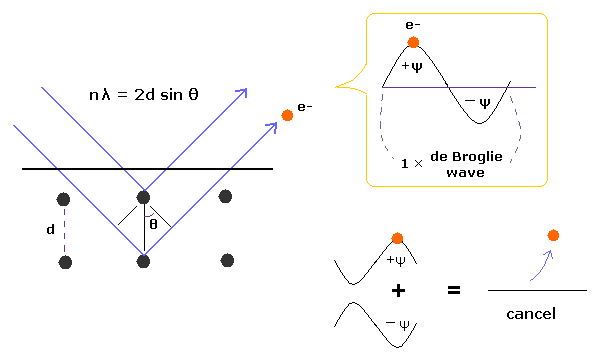In Davisson-Germer experiment, they accelerated electrons by electric fields and made them reflected by nickel crystal.
de Broglie wavelength of an electron is gotten from the electric field strength and interference pattern of a single electron.

Experimental results agreed with de Broglie relation.
So de Broglie waves themselves are realistic concepts, though quantum mechanics avoids commenting it.

This experiment showed an single electron has wave-like property, in which the opposite wave phases cancel each other, and expel the electron.

## One de Broglie wavelength consists of low and high pressure areas.

(Fig.5)  One de Broglie wavelength of electron.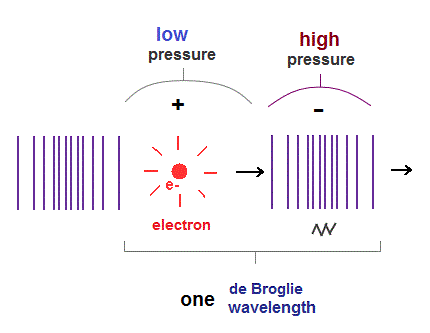When an electron moves, electric fields (= E ) around the electron also moves with it.
So this spreading electric field causes de Broglie waves, which can be confirmed by Davisson-Germer experiment.

We can naturally think this de Broglie wave is a kind of longitudinal waves through some medium.
If so, double slit interference can be explained by this "real" de Broglie waves, NOT by "fantasy" many-worlds.

Longitudinal wave consists of "low" (= sparse ) and "high" (= dense ) pressure areas.
It is thought that an electron pushes the field around it toward "dense" area, and is moving with "low" pressure area.

## de Broglie wavelength depends on the magnitude of momentum.

### [ As an electron moves "faster", de Broglie wavelength becomes "shorter". ]

(Fig.6)   Relation between electron's velocity (= v ) and de Broglie wavelength (= λ ).According to de Broglie theory, the electron's wavelength (= λ ) is equal to λ = h/mv, where h is Planck constant, "m" is mass, and "v" is "velocity".

So as an electron moves faster, its de Broglie wavelength becomes shorter.   "Momentum" means "impluse (= Ft )", so bigger momentum can apply more pressure on surrounding field, and compress it.

## Pressures of "momentum" and "field" are balanced → de Broglie wavelength.

### [ Total energy is conserved → particle's velocity and wavelength remain the same. ]

(Fig.7)  "Field" is compressed enough to maintain its shape, resisting momentum.When an electron with mass "m" is moving at a velocity of "v", its momentum is "mv".
In Fig.7 left, "field" is only slightly compressed, which pressure is lower than electron's momentum.

In this case, the stronger momentum pressure causes "field" to be more compressed, until their forces are equal (= balanced ) to each other (= Fig.7 right ).

Once the pressures from particle's "momentum" and compressed field are balanced, this field distortion is kept as "de Broglie wave", meaning its potential energy ( like spring ) remains the same.

Total energy must be conserved, so if the field potential (= distortion ) energy remains the same (= de Broglie wavelength is unchanged ), particle's kinetic energy is also conserved, keeping "uniform motion".

## "Dense" proton can compress field more than "thin" electron.

### [ "Slow" proton with bigger mass generates the same de Broglie wavelength as "fast" electron. ]

(Fig.8)  Proton's mass (= M ), velocity (= V ).  Electron's mass (= m ), velocity (= v ).A proton is about 1840 times heavier than an electron, so even when the proton is moving much slower than the electron, their momentums can be the same ( MV = mv ).

In this case, their de Broglie wavelengths are also the same ( λ = h/MV = h/mv ).
Larger mass of a proton means the density of "positive" field is much higher than "nagetive" field of an electron.

As a result, denser proton exerts higher pressure on surrounding "field", even if its speed is lower than an electron.

Considering "dense (= + )" and "thin (= - )" fields attract each other, Coulomb forces can be explained by the difference in field density.  ( the same charges, "dense" - "dense",  "thin" - "thin" repel each other. )

## An integer times de Broglie wavelength orbit is stable.

### [ Two de Broglie waves fitting each other in the same phase are stable. ]

(Fig.9)  Wave phases at both ends fit each other = n × de Broglie wavelength.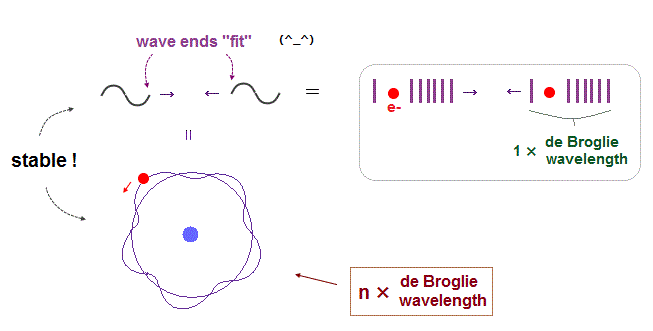As seen in Bohr model, when an orbit is an integer (= n ) times de Broglie wavelength, their wave phases are consistent with each other, avoiding destructive interference, so stable.

In this page, we proved that Schrodinger equation also satisfies this "n × de Broglie wavelength" rule, tacitly, which is the reason both Bohr and Schrodinger hydrogens give the same energy levels.

Considering electron's de Broglie wave is one of longitudinal waves, their wave phases (= repeat of high and low pressure regions ) harmonize with each other, keeping stable wave states.

(Fig.10) de Broglie wave ends fit.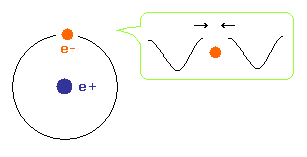To avoid cancelling de Broglie wave, both ends of electron's wave in hydrogen atom need to agree with each other with respect to wave phases.

## "Photon" is a fictitious particle without clear physical shape.

### [ Quantum mechanics cannot answer "What are the "shape" and size of a photon ?" ← unreal. ]

(Fig.11)   Photodetectors just see amplified "electric" current, NOT a photon itself.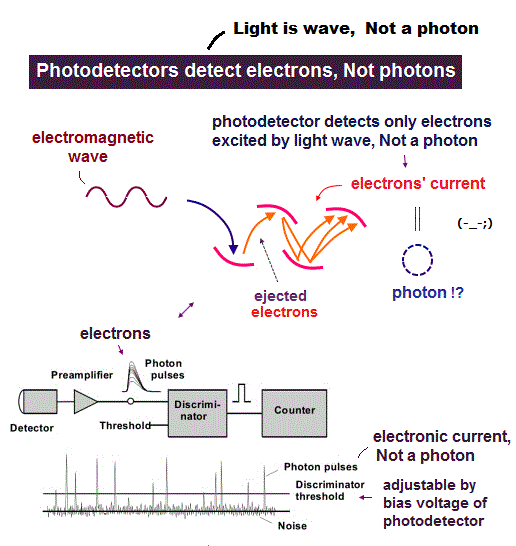Though the media and textbooks repeatedly use the word of "photon", those media said nothing about the shape and size of a single photon.

Feynman QED just shows abstract math symbols without physical shape as a "photon" ( see this p.2, this ).   For example, a photon is created (= a ), or annihilated (= a ). That's all. NOT physics.

A single photon detector just measures amplified "electrons", cannot see a photon itself. See this, this p.4   This photodetector depends on photoelectric effect, in which an electron is ejected by a photon (= light ).

Though textbooks ( this, this ) say photoelectric effect depending on light "frequency" proves photon "particle", the frequency (= c/wavelength ! ) clearly means wave nature of light.

## Why is electromagnetic wave emitted as "transverse" wave ?

### [ When electron moves to lower energy level, it emits "transverse" waves. Why ? ]

(Fig.12)  Emitted electromagnetic wave is always "transverse" wave !It is known that electromagnetic wave is "transverse" wave, consisting of electric and magnetic fileds, they insist.

These electromagnetic waves (= light or photon ? ) are emitted ( or absorbed ), when each electron moves to lower ( higher ) energy levels.

Then, why are these electromagnetic waves always "transverse" ( NOT longitudinal ) waves ?

## Both "circular" and "elliptical" orbits obey n × de Broglie wavelength.

(Fig.13) From "circular" Bohr model to "elliptical" Sommerfeld model.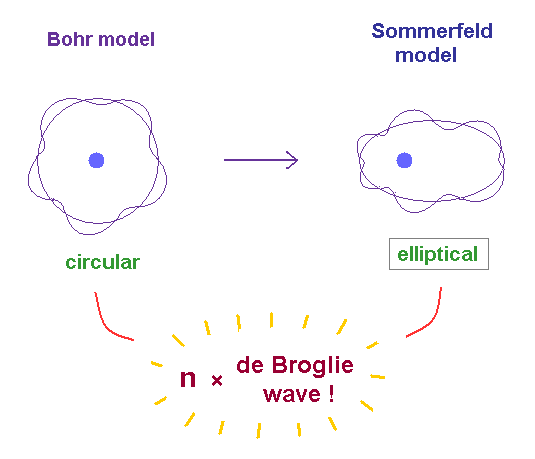In 1916, Arnold Sommerfeld extended Bohr's circular orbit to "elliptical" one.
Sommerfeld elliptical orbit also satisfies an integer number of de Broglie wavelength.

This condition is expressed as Sommerfeld quantization rule in both radial and angular directions, as shown on this site (p.119) and this site (p.12).

In these quantum theories ( including Schrodinger equation ), stable de Broglie waves are indispensable for stable orbits.  Between them, electromagnetic transverse waves are emitted.

## "Crash" between de Broglie waves causes "transverse" electromagnetic wave.

### [ When orbits are NOT an integer de Broglie wavelength, their de Broglie waves "crash". ]

(Fig.14)  Crash between de Broglie waves causes "transverse" EM waves.When each orbit is NOT an integer times de Broglie wavelength, the phases of these de Broglie waves do NOT fit each other, so "crash" between de Broglie waves happens.

When "upper" and "lower" de Broglie waves crash into each other, electromagnetic waves are emitted to the right ( or left ), which becomes "transverse" wave, naturally.

## Why "longitudinal" waves are NOT allowed in transitions ?

### [ "Pressures" from two de Broglie waves "prevent" emission of longitudinal waves. ]

(Fig.15)  "Upper" and "lower" wave pressures prevent longitudinal emission.If "longitudinal" wave is emitted instead of "transverse" wave in energy transitions, its direction is parallel to two "crashing" de Broglie waves (= upper and lower in Fig.15 ).

But thinking commonsensically, this "longitudinal" emission is impossible.
Pressures from upper and lower de Broglie waves prevent longitudinal waves from being emitted in "parallel" direction.

Only directions perpendicular to these two de Broglie waves are allowed, when electromagnetic waves are emitted, which naturally becomes "transverse" wave.

As shown in helium, when two de Broglie waves cross perpendicularly, their phases are independent and NOT interfering with each other, which does NOT cause "crash".

## Crash beween two de Broglie waves naturally causes transverse wave.

### [ "Transverse" direction is an outlet for energy from "crashed" de Broglie waves. ]

(Fig.16)  Electromagnetic wave is emitted in "pressure-free" directions  ← "transverse".When two de Broglie waves crash into each other from "upper" and "lower" directions, their energies are emitted in horizontal (= transverse ) direction, which has NO de Broglie wave pressures.

This emitted electromagnetic wave takes over "oscillating (= up and down ) energies" from de Broglie waves to satisfy total energy conservation.

This oscillating direction in "crashed" de Broglie waves is the origin of transverse wave inside emitted electromagnetic wave, as shown in Fig.16.

In circular orbits, an electron is periodically moving in closed system, so its de Broglie wave is oscillating up and down ( or right and left ), meaning the average momentum is zero in transverse direction.

## Electromagneic wave is a mixture of "transverse" and "longitudinal" wave.

(Fig.17)  True figure of electromagnetic wave ↓  transverse and longitudinal mixed.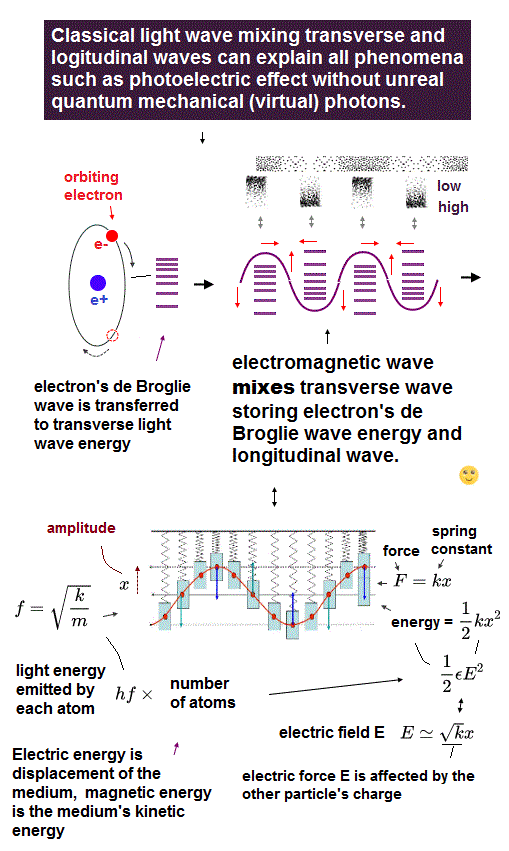As seen in Compton scattering, the electromagnetic wave also has the property of de Broglie wave in the direction of travel (= to the right in Fig.16 ).

Crash between two de Broglie waves is thought to push electromagnetic waves in the right direction, as a form of "longitudinal" de Brolgie wave.

So the electromagnetic wave has both "transverse" (= original de Broglie wave oscillating direction ) and "longitudinal" (= pushed out ) directions inside one wave.

Due to the balance in the field pressure, high-pressure area in transverse direction is low-pressure in longitudinal direction. They are alternating.

In Maxwell equation, changing electric field originally means the electric current (= de Broglie wave ). This page proved magnetic field is generated by de Broglie waves, which can be applied to this electromagentic wave.

## Why an electron cannot absorb "longitudinal" waves ?

### [ Only "transverse" electromagnetic wave is absorbed in electron's energy transition. Why ? ]

(Fig.18)  "Longitudinal" waves are NOT absorbed. Why ?If each electron can absorb "longitudinal" waves in addition to transverse electromagnetic waves, we can also see "longitudinal" wave with various colors through chemical reaction inside eyes.  But we cannot.

This fact shows there are some reasons why each electron in energy transition can absorb only "transverse" electromagnetic wave, like in case of "emission"

## Two parallel de Broglie waves "crash" → "longitudinal" waves are NOT absorbed.

### [ Overlapping of two "different" de Broglie waves causes them to avoid each other. ]

(Fig.19)  "Longitudinal" waves "avoid" each other !To transfer the energy of one "longitudinal" wave to another de Broglie (= longitudinal ) wave of electron, they must crash into each other in the parallel direction.

The wavelength, phases and velocities are different between de Broglie waves with different "masses", which causes "crash" between two different de Broglie waves.

As a result, two different "longitudinal" ( de Broglie ) waves tend to avoid each other to prevent "crash".  This is the reason why "longitudinal" wave cannot be absorbed into an electron.

Besides this reason, when a particle with different mass is absorbed into other particles, it cannot satisfy both energy and momentum conservation, as seen in antiparticle generation.

## "Transverse" electromagnetic waves can be absorbed "safely".

### [ "Transverse" wave can "approach" de Broglie waves, NOT disturbing them. ]

(Fig.20)  "Transverse" wave can approach de Broglie wave "perpendicularly".On the other hand, transverse electromagnetic waves can approach other electron's de Broglie waves from the perpendicular direction.

As shown in helium case, "perpendicular" means two de Broglie waves can approach each other independently ( in phase ), NOT disturbing other waves.

After they approach and cross each other, transverse oscillation energies stored in electromagnetic waves are transferred and absorbed into electron's de Broglie wave, safely.

This is thought to be the main reason why each electron can absorb only "transverse" ( NOT longitudinal ) electromangetic wave.

## Why "neutrino" is always "light-speed", and can pass through the earth ?

### [ Unstable Muon is just a "composite" particle of an electron and "neutrino" ! ]

(Fig.21)  Unstable muon (= particle ? ) decays into an electron and neutrino instantly !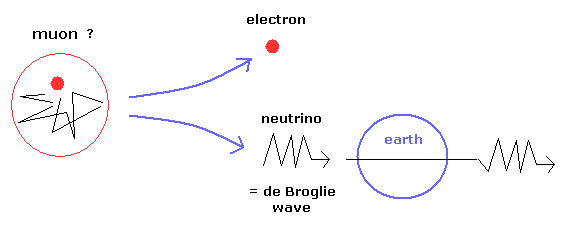Unstable muon's lifetime is very short (= about 2.2 microsecond ), it decays into an electron and neutrino instantly.  So, calling muon "elementary particle" is very unreasonable.

We can naturally think this transient muon is a "composite" state consisting of an electron and neutrino.  It is known that this neutrino always travels at light speed.

And this neutrino can pass through the earth, different from ordinary "particles".
From these two properties, we can naturally think this neutrino is de Broglie wave oscillation, NOT a particle.

## Oscillations in the Same medium cause "constant" light speed.

### [ Neutrino is "longitudinal" wave, light is "transverse" wave in the "same" medium.  ← light speed c ! ]

(Fig.22)  Why "neutrino" and "light" always travel at light-speed c ?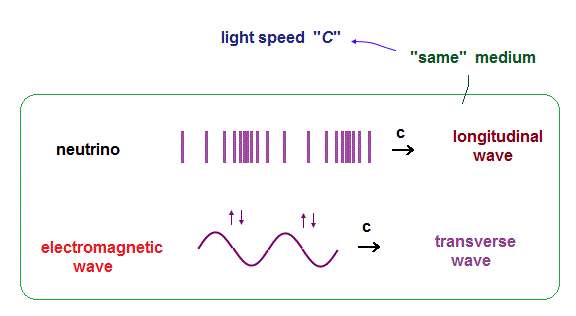It is known both neutrino and electromagnetic wave always travel at light-speed c.
"Constant" speed means these things are some oscillations travelling in the same common medium.

## Why an electron cannot absorb "neutrino" in transition ?

### [ "Longitudinal" neutrinos crash and avoid other electron's de Broglie waves ! ]

(Fig.23)  Neutrino can cause only "Compton" scattering.If "neutrino" can be absorbed into an electron in energy transition, we can "see" (= chemical reaction inside eyes ) these neutrinos like electromagnetic waves.  But we cannot.

As I said in this section, if we think neutrino is one of "longitudinal" de Broglie waves, two different de Broglie waves tend to crash and avoid each other in parallel direction.

As a result, these neutrinos can pass (= avoid ) other particles de Broglie waves, NOT interacting with each other.  Neutrino can interact with electrons only through Compton-like scattering.

## Only transverse electromagnetic wave can be "absorbed".

### [ "Perpendicular" direction does NOT disturb other de Broglie waves ! ]

(Fig.24)  Transverse wave can approach electrons, "perpendicularly" and safely.Different from longitudinal neutrino, transverse electromagnetic wave can approach other electron's de Broglie waves from the "perpendicular" direction.

Its energy is stored in "transverse" direction, so after approaching and crossing electron's de Broglie waves, this stored oscillating energy is transferred to other electrons, safely.

This is the true mechanism of light "absorption" and photoelectric effect.

## Why electromagnetic wave is so susceptible to environement ?

### [ Electromagnetic waves contains the opposite oscillations in small area ← unstable.]

(Fig.25)  If "transverse" oscillation disappears, longitudinal component also disappears.Different from neutrino, the electromagnetic waves are very susceptible to environment.
One of reasons is easy absorption into electrons, because they can cross each other perpendicularly.

As shown in Fig.25, inside electromagnetic waves, both "transverse" and "longitudinal" directions contains the opposite oscillations in small areas.

So when transverse oscillation disappears ( by absorption ), the opposite longitudinal oscillations cancel each other, and disappears, too.

As seen in balanced field pressures, even when only transverse oscillations are disturbed and slowed down, it instantly affects "longitudinal" oscillation, to keep their field balance.

Even when longitudinal wave can pass through other waves perpendicularly and safely, "transverse" oscillation may be caught by them.   It can explain the light "slow-down" inside various medium such as water,

## Magnetic force between electric currents can be explained by de Broglie waves.

(Fig.26)  Magnetic (= Lorentz ) force between two wires = de Broglie waves.As shown on this site and this, according to Lorentz magnetic force, two wires carrying electric currents in the same direction attract each other.  And the opposite currents repel each other.

The electric current is the flow of ( moving ) charged particles (= electron ).
Moving electrons generate their de Broglie waves, so it is clear this de Broglie wave has something to do with magnetic force !

## Opposite electron's de Broglie waves (= currents ) repel each other.

(Fig.27)  The opposite de Broglie waves (= currents ) have the "opposite" oscillating velocities.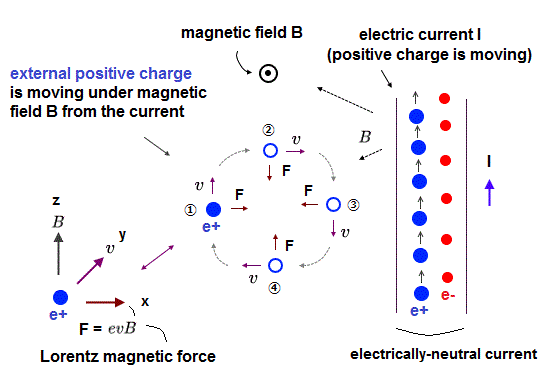As I said, electron's de Broglie waves propagating in the opposite directions contain the opposite oscillating fields in the same-phase areas, so these opposite waves crash and repel each other.

The electric currents are moving electrons (= de Broglie waves ).
So the repulsive de Broglie waves express the electric currents repelling each other by magnetic forces.

## de Broglie waves (= currents ) in the "same" direction "attract" each other.

(Fig.28)  de Broglie waves in the same direction contain the same oscillation.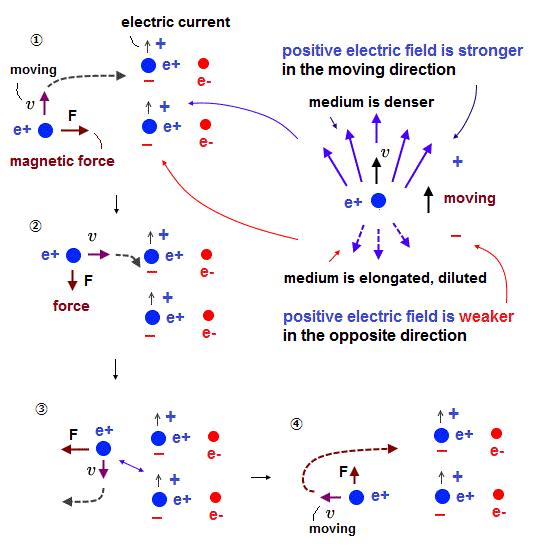When two de Broglie waves propagate in the same direction, their oscillations are the same and harmonize with each other (= constructive interference ).

As a result, two de Broglie waves ( electric currents ) in the same direction attract each other, which is thought to be Lorentz "magnetic" force.  It is similar to Magnus effect.

## Reason why helium shows no magnetic property.

(Fig.M1)  Hydrogen and helium de Broglie wave models.It is known that one-electron hydrogen has magnetic moment (= Bohr magneton ), and two-electron helium shows no magnetic property.

Spin-spin magnetic interaction is too weak to explain Pauli exclusion principle.  So spin is unreal.
Here we use "realistic" de Broglie wave model of helium to explain it.

## "Opposite" phases in the "same" direction cancel each other.

(Fig.M2)  Opposite wave phases in the same direction cancel magnetic in He.For two electrons' de Broglie waves to avoid canceling each other, they have to be perpendicular to each other in "realistic" helium model.

e1 ( e2 ) electron and e2 ( e1 ) opposite wave phase are moving in the same direction, as shown in Fig.M2.  So these opposite phases ( in the same direction ) cancel each other at the point of a distant detector.

As I said, magnetic field is related to de Broglie wave (= electron's movement ), so these canceled ( from a distant place ) wave phases show "average zero" magnetic property of helium.

## "Fractional" charge quark is too good to be ture.

### [ Fractional charge quark can NEVER be isolated. Why the sum of them becomes just +e ? ← Too good ! ]

(Fig.29)  "Independent" protons, electrons are just the "same" amount !?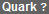It is said that the proton is composed of three quarks with fractional charges.
But this fractional charges ( +2/3e, -1/3e ) can NEVER be isolated, so quark has NO real evidence.  See this and this.

They just jumped to a conclusion that Jets (= only electrons and lights can be detected ) may be quark or gluon. In standard model, quarks are just meaningless math symbols with NO physical shapes.

Originally, fractional charges such as +2/3 (= up ) and -1/3 (= down ) quarks are completely independent from (= NO relation with ) electrons.  ( Why 3/4e, 4/7e is impossible ? )

But the sum of them (= 2 × up + 1 × down quarks ) becomes just +e charge proton, which number is "accidentally" just the Same as -e charge electron in the universe !  ← Too good to be true!

On the other hand, the number of positron, which is said to be the partner of electron, is much smaller than electrons, and unstable antiparticles disappear instantly (= within 10-12 seconds ! ).

And these antiparticles disobey basic physical law such as total momenum conservation.
So standard model based on these quarks lacks reality.

## Why proton and electron charges are exactly the same amount ?

### [ The current quantum mechanics cannot answer why the universe contains the same amount of ±charges ! ]

(Fig.30)  Imbalance in "field pressure" causes plus, minus charges.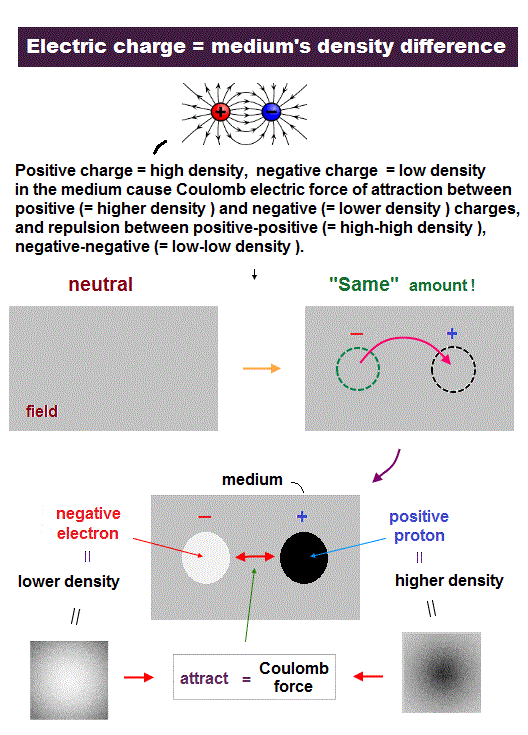Quantum mechanics cannot answer "why the universe contains exactly the same amount of plus, minus charges" as protons and electrons.  And they have NO definite answer, "why a proton is much heavier than an electron".

If we suppose Coulomb force is caused by the difference in field pressures (= density ), we can naturally answer all these basic questions.

In Fig.30 upper, some amount of field matters are transferred to another places.
This causes a pair of "thin" area with lower pressure, and "dense" area with "higher" pressure in the field.

If the field matters are unevenly distributed, they try to go back to the uniform density, which is the origin of Coulomb attraction.  "Dense" proton is naturally heavier than "thin" electron.

Due to pressure balance, the combinations of "dense-dense (= +, + )" and "thin-thin (= -, - )" areas repel each other, which is why the same charges repel each other in Coulomb forces.

## "Dense" proton is much heavier than "thin" electron.

### [ Total momentum conservation causes an light electron to move much faster. ]

(Fig.31)  An proton is 1840 times heavier (= denser ) than an electron.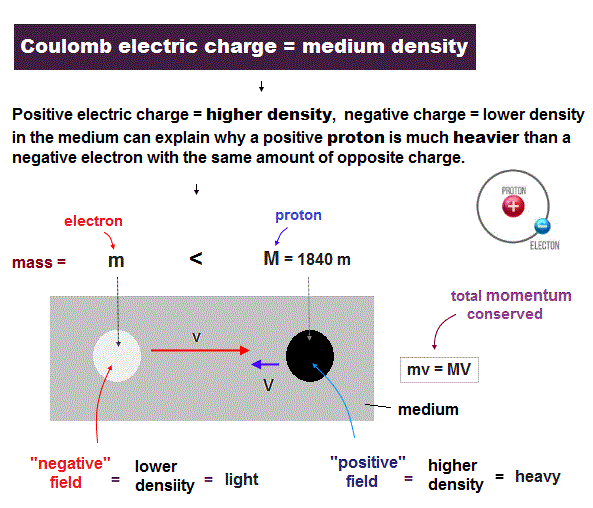It is known that a proton is about 1840 times heavier than a electron.  If we suppose, a proton (= positive field ) is 1840 times denser than an electron (= negative field ), this big difference in mass can be explained.

Due to imbalance in field densities (= "positive" is dense, "negative" is thin ), the opposite charges attract and start to move towards each other.

Total momentum must be conserved due to the law of action and reaction.
So a "light" electron must move much ( 1840 × ) faster than a heavy proton during the same time.

## Momentums are equal → proton and electron have the same de Broglie wavelength.

(Fig.32)  The same momentum causes the same de Broglie wavelength.According do de Broglie theory, de Broglie wavelength (= λ ) is determined by the particle's momentum ( λ = h/p ), irrespective of particle's kinds.

So even when a proton's velocity is lower, it can cause the same de Broglie wavelength (= distortion of the field ) as an electron, if they have the same momentum.

Because "denser" proton can distort the surrounding field as much as "thin" electron, even when proton is moving more slowly.

## Potential energy is conserved → Each charge (= field imbalance ) is conserved.

(Fig.33)  "Thin" area (= negative ) is kept due to conservation of total energy.Of course, total energy must be conserved, so once "distortion" of the field is formed, its imbalance (= potential energy ) is conserved.  As a result each positive and negative charges are conserved.

In Fig.33 left, some field particle is attracted toward "thin" negative field, and accelerated (= kinetic energy increases ).  It moves until it reaches the same potential energy (= imbalance ), keeping negative charge .

(Fig.34)  Positive charge is conserved due to energy conservation.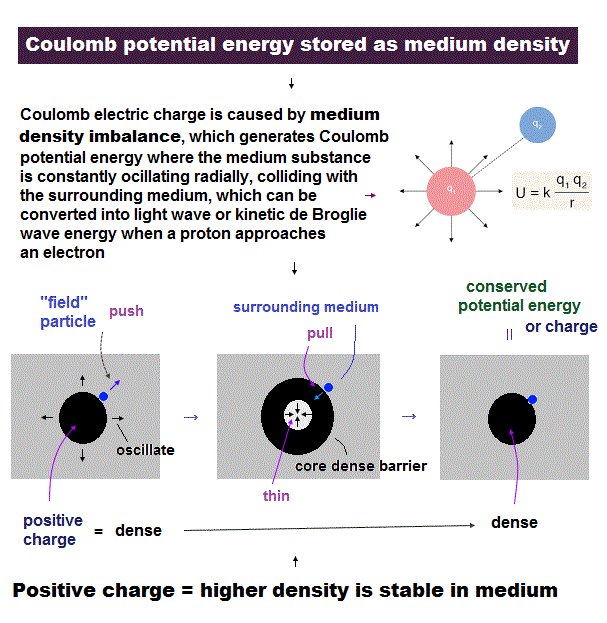Also in the positive proton, the conservation of total ( potential ) energy keeps the original amount of charge.  In Fig.34 left, some field particle is pushed outward by high-pressure positive field.

This force is changed into particle's kinetic energy, and it continues until the pressure in the center is lower enough to get them back to the original places, due to conservation of potential energy.

This is the mechanism in which each positive charge (= +e ) is conserved.
The point is, when field becomes dense enough to cause "+e", the surrounding field cannot enter the central part, even when the field is expanded in Fig.34 middle.

If total charge is smaller than this +e, the surrouding field matters can enter the central part, when the proton is expanded, which tears up a proton into scattered pieces (= potential energy splits into smaller kinetic energies ).

This theory can explain why the nature adopts only "±e" as "quantum charge".

## Reason why "frequency" is proportional to energy in waves.

(Fig.35) de Broglie relation.According to de Broglie relation, the momentum ( p = mv ) is expressed as ( p = h/λ ).
Here, p = momentum, m = mass, v = velocity, h = Planck constant, λ = wavelength.

As shown on this site, total energy (= E ) of electromagnetic wave is linearly proportional to frequency (= f ) through the relation of E = hf, which is confirmed in photoelectric effects.

Surprisingly, in any other particles such as electrons and protons, their frequency is proportional to their total energy, if de Broglie relation is satisfied !  Here we explain it.

## Frequency is "proportional" to energy also in electrons.

### [ Besides light, an electron's energy is also proportional to its frequency. ]

(Fig.36)  Electron is slower ( v → 1/2 v ), its wavelength becomes longer ( λ → 2λ ).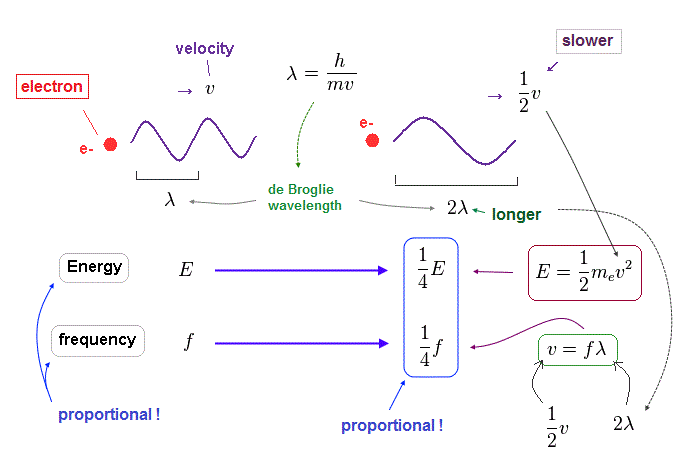When an electron's velocty (= v ) becomes half (= 1/2 ), its total ( kinetic ) energy (= E ) becomes 1/4 of the original value, because E is proportional to a square of velocity ( E = 1/2 mv2 ).

According to de Broglie relation ( mv = h/λ ), the electron's wavelength becomes twice ( λ → 2λ ), when the velocity becomes half.

Considering wave relation ( v = fλ ), the electron's frequency (= f ) becomes 1/4 of the original value in this case.
As you see, also in electron's de Broglie wave, the energy is proportional to frequency ( E/f = constant ) !

## Also in proton, its energy is proportional to wave frequency.

### [ When the momentums of "light" electron and "heavy" proton are the same .. ]

(Fig.37) Momentum ( p = h/λ ) is constant.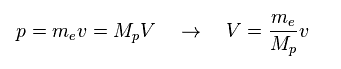Here we think about the case when the momentum (= p ) is common in both of "light" electron and "heavy" proton.
So through de Broglie relation ( λ = h/p ), their wavelengths (= λ ) are the same, too.

Due to heavier mass ( Mp ) of proton, the velocity (= V ) of proton becomes much lower than that (= v ) of electron.
So the total kinetic energy ( E = 1/2 MpV2 ) of proton is much smaller than electron (= 1/2 mv2 ).

(Fig.38) Energy (= E ) is proportional to frequency (= f ) in electron and proton.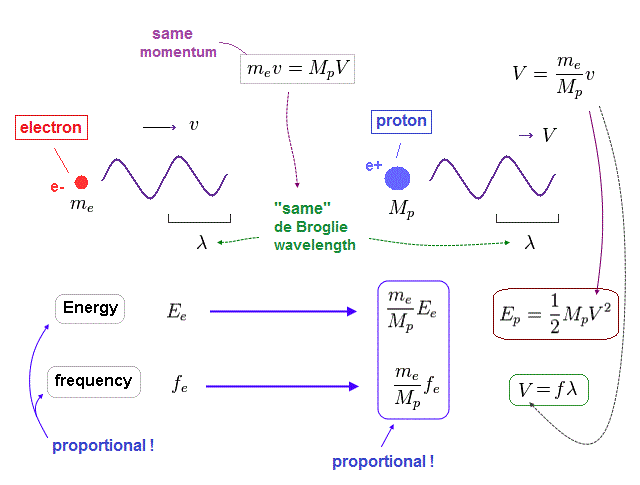Again, through the wave relation of v = fλ, we can find the proton's frequency.
As shown in Fig.38, between proton and electron, the relation of energy (= E ) proportional to frequency (= f ) holds true.

So not only in electromagnetic wave but also in electrons and protons, the frequency has an important meaning to determine their total energy.

(Fig.39) Total energy in electron and proton.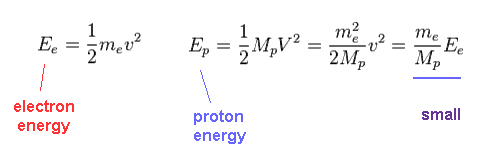Using Fig.37, we find the proton's kinetic energy is much smaller than electron due to its large mass, even when their momentums are the same.

(Fig.40) Ratio of electron's to proton's masses.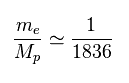Proton (= Mp ) is much heavier ( 1836 × ) than electron's mass (= me ).

(Fig.41) Frequency = velocity / wavelength.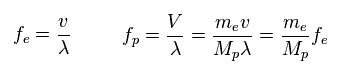Through wave relation, we find the proton's frequency becomes much smaller than electron.
( Here the wavelengths ( momentums ) of electron and proton are the same. )

## Light's frequency, energy and its speed (= c )

(Fig.42) Light's energy is proportional to its frequency, too.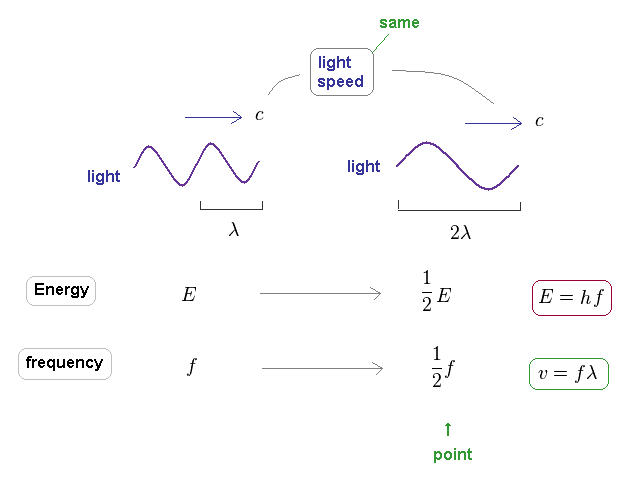It is known that light's energy is also proportional to its frequency through the relation of E = hf.
The important point is that the speed of the light is always constant "c", different from other particles.

This means there is NO concept such as "acceleration" in light ( and neutrino ).
When electron's ( or proton's ) wavelength changes, both of its velocity and momentum changes.

So the electron's frequency is inversely proportional to a square of wavelength, as shown in Fig.36.
On the other hand, due to neglecting light speed change, the light frequency is just inversely proportional to its wavelength.

As you see, de Broglie wavelength ( λ = h/p ) expresses "contraction" ( or expansion ) of the field by the momentum (= impulse ), compared to its equilibrium state, irrelevant to the velocities of various particles.

## Relation between electron's and emitted light's frequencies.

(Fig.43)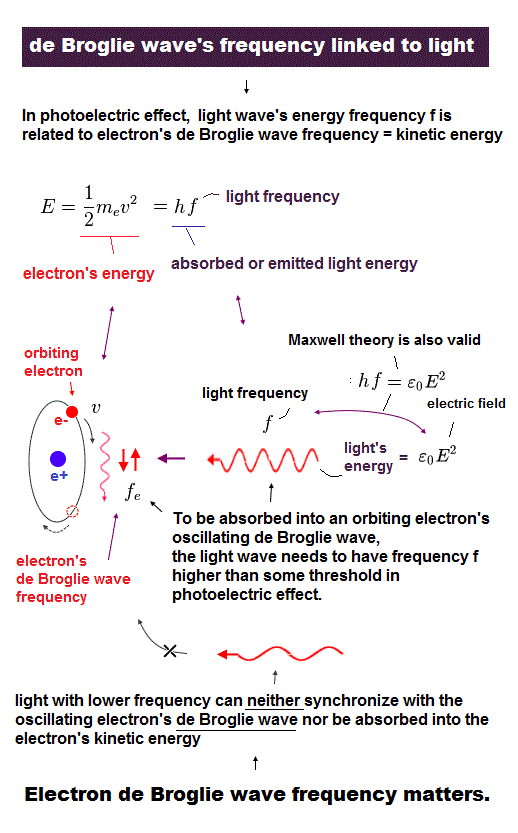Here we investigate the relation between electron's frequency (= fe ) and emitted light's frequency (= f ).
In Fig.43, total kinetic energy of electron (= 1/2mv2 ) is equal to light's energy (= hf ) emitted from the electron.

(Fig.44)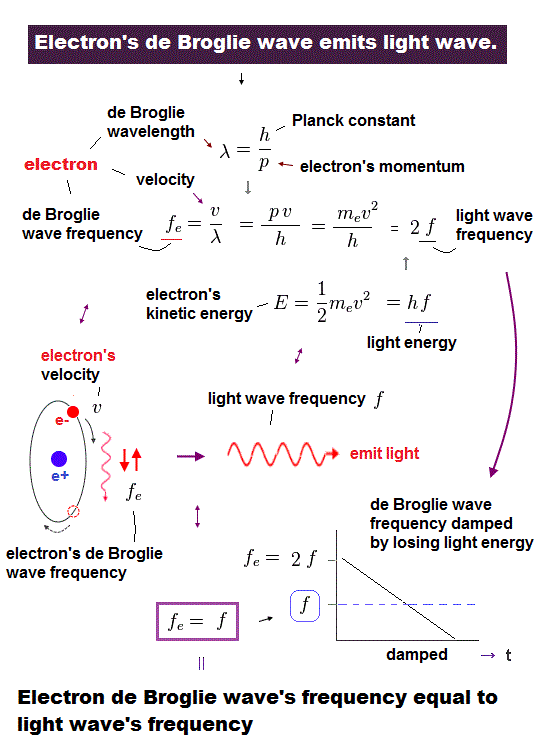Using wave relation ( v = fλ ) and de Broglie relation ( λ=h/p ), we find that the electron's ( de Broglie ) frequency (= fe ) is two times bigger than the emitted light frequency (= f ).

## Average frequency of "damped" electron's oscillation is equal to emitted light (= f ).

(Fig.45) Oscillating electron emits light and gradually loses its energy.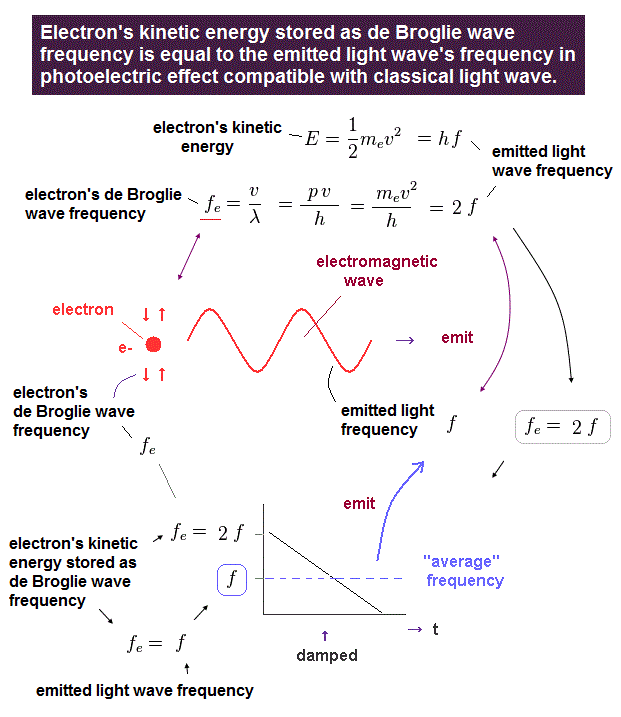In Fig.45, we think about the case in which oscillating electron gradually emits light and loses its kinetic energy.
The initial electron's frequency is equivalent to 2 × f (= emitted light frequency ), as shown in Fig.44.

The more light the electron emits, it loses more energy, and its oscillation becomes slower ( to be zero ).
It means the average electron's frequency becomes half of the original value (= 1/2 × fe = f ) .

As a result, we can prove there is an important relation between electron's and emitted light frequencies.  Planck constant (= h ) connects light and electron.

## Frequencies of two de Broglie waves and emitted light.

(Fig.46) Transition from excited ( n = 2, f=4 ) state to ground state ( n =1, f = 2 ).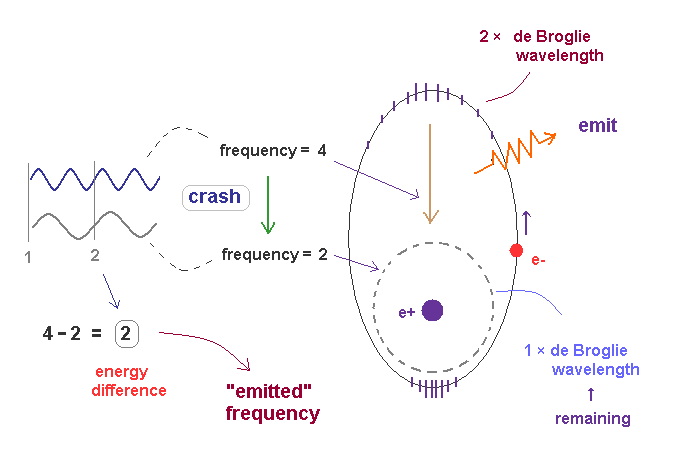In Fig.46, the excited state of 2 × de Broglie wavelength returns to ground state (= 1 × de Broglie wavelength ).
Accelerated by Coulomb force, the final frequency of de Broglie wave of excited electron becomes f = 4.

We can think the afterimage of stable ground state de Broglie wave remains during excitation, and it is supposed to be f = 2.
These two de Broglie frequencies before and after transition are different, so they don't fit each other.

The crash between these two de Broglie waves causes swell with the frequency ( 4-2 = 2 ), which is the difference between two states.

The excited de Broglie wave is attenuated, while it emits light.
So the average frequency of emitted light becomes half of 2 (= 1 ), as is explained in Fig.45.

## Virial theorem → kinetic energy (= frequency ) is absolute value of total energy.

(Fig.47)  Transition from excited ( n = 2, f=2 ) state to ground state ( n =1, f = 4 ).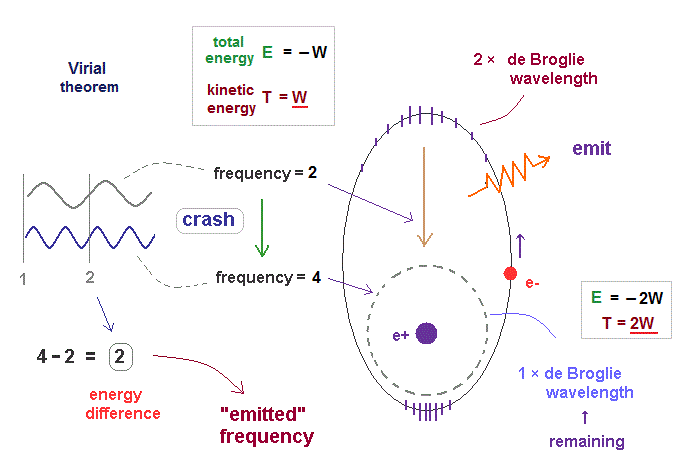In fact, according to Virial theorem, kinetic energy T is the absolute value of total energy E ( T = |E| ).
As I said in Fig.36, electron's frequency (= f ) is proportional to its kinetic energy.

So the electron frequency in the upper energy level is lower than the lower level, like their kinetic energies. Also in this case, the frequency difference becomes 4 - 2 = 2, like Fig.46.

So the difference in total energies is just equal to the difference in kinetic energies (= frequency ) in these bound state orbits, which can explain photoelectric effect correctly in the realistic way. See this.2015/5/17 updated. Feel free to link to this site.